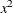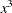#### CLASS Variable Parameterization

Consider a model with one CLASS variable `A` with four levels: 1, 2, 5, and 7. Details of the possible choices for the PARAM= option follow.

EFFECT

Three columns are created to indicate group membership of the nonreference levels. For the reference level, all three dummy variables have a value of –1. For instance, if the reference level is 7 (REF=7), the design matrix columns for `A` are as follows.

Design Matrix

A

A1

A2

A5

1

1

0

0

2

0

1

0

5

0

0

1

7

–1

–1

–1

For CLASS main effects that use the EFFECT coding scheme, individual parameters correspond to the difference between the effect of each nonreference level and the average over all four levels.

GLM

As in PROC GLM, four columns are created to indicate group membership. The design matrix columns for `A` are as follows.

Design Matrix

A

A1

A2

A5

A7

1

1

0

0

0

2

0

1

0

0

5

0

0

1

0

7

0

0

0

1

For CLASS main effects that use the GLM coding scheme, individual parameters correspond to the difference between the effect of each level and the last level.

ORDINAL

Three columns are created to indicate group membership of the higher levels of the effect. For the first level of the effect (which for `A` is 1), all three dummy variables have a value of 0. The design matrix columns for `A` are as follows.

Design Matrix

A

A2

A5

A7

1

0

0

0

2

1

0

0

5

1

1

0

7

1

1

1

The first level of the effect is a control or baseline level.

For CLASS main effects that use the ORDINAL coding scheme, the first level of the effect is a control or baseline level; individual parameters correspond to the difference between effects of the current level and the preceding level. When the parameters for an ordinal main effect have the same sign, the response effect is monotonic across the levels.

POLYNOMIAL | POLY

Three columns are created. The first represents the linear term (x), the second represents the quadratic term (), and the third represents the cubic term (), where x is the level value. If the CLASS levels are not numeric, they are translated into 1, 2, 3,according to their sort order. The design matrix columns for `A` are as follows.

Design Matrix

A

APOLY1

APOLY2

APOLY3

1

1

1

1

2

2

4

8

5

5

25

125

7

7

49

343

REFERENCE | REF

Three columns are created to indicate group membership of the nonreference levels. For the reference level, all three dummy variables have a value of 0. For instance, if the reference level is 7 (REF=7), the design matrix columns for `A` are as follows.

Design Matrix

A

A1

A2

A5

1

1

0

0

2

0

1

0

5

0

0

1

7

0

0

0

For CLASS main effects that use the REFERENCE coding scheme, individual parameters correspond to the difference between the effect of each nonreference level and the reference level.

ORTHEFFECT

The columns are obtained by applying the Gram-Schmidt orthogonalization to the columns for PARAM=EFFECT. The design matrix columns for `A` are as follows.

Design Matrix

A

AOEFF1

AOEFF2

AOEFF3

1

1.41421

–0.81650

–0.57735

2

0.00000

1.63299

–0.57735

5

0.00000

0.00000

1.73205

7

–1.41421

–0.81649

–0.57735

ORTHORDINAL | ORTHOTHERM

The columns are obtained by applying the Gram-Schmidt orthogonalization to the columns for PARAM=ORDINAL. The design matrix columns for `A` are as follows.

Design Matrix

A

AOORD1

AOORD2

AOORD3

1

–1.73205

0.00000

0.00000

2

0.57735

–1.63299

0.00000

5

0.57735

0.81650

–1.41421

7

0.57735

0.81650

1.41421

ORTHPOLY

The columns are obtained by applying the Gram-Schmidt orthogonalization to the columns for PARAM=POLY. The design matrix columns for `A` are as follows.

Design Matrix

A

AOPOLY1

AOPOLY2

AOPOLY5

1

–1.153

0.907

–0.921

2

–0.734

–0.540

1.473

5

0.524

–1.370

–0.921

7

1.363

1.004

0.368

ORTHREF

The columns are obtained by applying the Gram-Schmidt orthogonalization to the columns for PARAM=REFERENCE. The design matrix columns for `A` are as follows.

Design Matrix

A

AOREF1

AOREF2

AOREF3

1

1.73205

0.00000

0.00000

2

–0.57735

1.63299

0.00000

5

–0.57735

–0.81650

1.41421

7

–0.57735

–0.81650

–1.41421